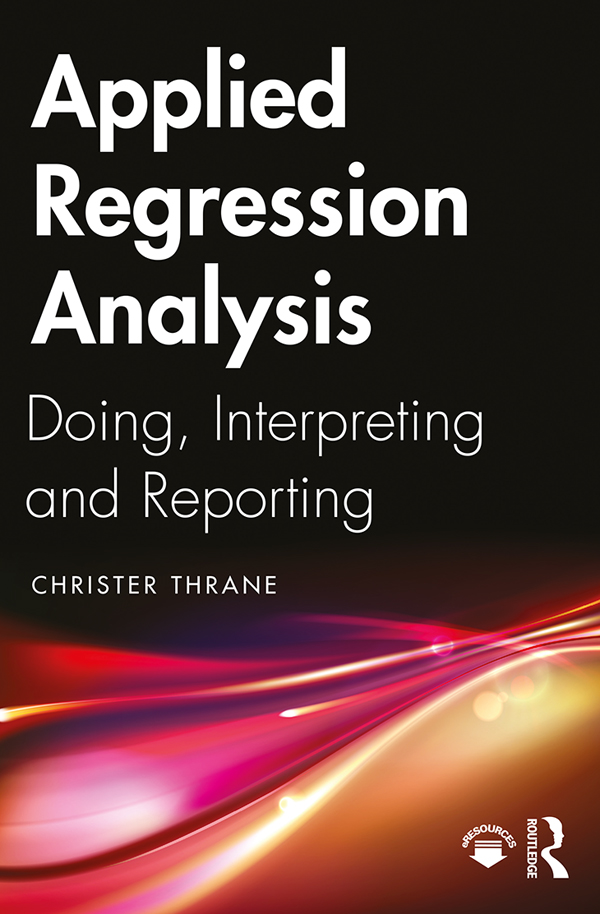# Applied Regression Analysis

## Doing, Interpreting and Reporting, 1st Edition

Routledge

192 pages | 65 B/W Illus.

Paperback: 9781138335486
pub: 2019-10-28
SAVE ~\$12.19
\$60.95
\$48.76
x
Hardback: 9781138335479
pub: 2019-10-30
SAVE ~\$32.00
\$160.00
\$128.00
x
eBook (VitalSource) : 9780429443756
pub: 2019-10-16
from \$30.48

FREE Standard Shipping!

### Description

This book is an introduction to regression analysis, focusing on the practicalities of doing regression analysis on real-life data.

Contrary to other textbooks on regression, this book is based on the idea that you do not necessarily need to know much about statistics and mathematics to get a firm grip on regression and perform it to perfection. This non-technical point of departure is complemented by practical examples of real-life data analysis using statistics software such as Stata, R and SPSS. Parts 1 and 2 of the book cover the basics, such as simple linear regression, multiple linear regression, how to interpret the output from statistics programs, significance testing and the key regression assumptions. Part 3 deals with how to practically handle violations of the classical linear regression assumptions, regression modeling for categorical y-variables and instrumental variable (IV) regression. Part 4 puts the various purposes of, or motivations for, regression into the wider context of writing a scholarly report and points to some extensions to related statistical techniques.

This book is written primarily for those who need to do regression analysis in practice, and not only to understand how this method works in theory. The book’s accessible approach is recommended for students from across the social sciences.

### Reviews

“This book finds a rare balance between applied statistical analysis and theory, giving students the confidence to apply regression analysis in their projects, while being aware of the potential pitfalls.” — Johan A. Elkink, Associate Professor in Social Science Research Methods, University College Dublin, Ireland

“This book provides a short and bright path to understand the meaning and usefulness of regression analysis. If you are a student or policy maker with limited econometrics skills this book equips you with the right and sufficient skills.” — Dr. Maty Konte, United Nations University

Part 1: The Basics 1. What is regression analysis? 2. Linear regression with a single independent variable 3. Linear regression with several independent variables: Multiple regression Part 2: The Foundations 4. Samples and populations, statistical uncertainty and testing of statistical significance 5. The assumptions of regression analysis Part 3: The Extensions 6. Beyond linear regression: Non-additivity, non-linearity and mediation 7. A categorical dependent variable: Logistic (logit) regression and related methods 8. An ordered (ordinal) dependent variable: Logistic (logit) regression 9. The quest for a causal effect: Instrumental variable (IV) regression Part 4: Regression Purposes, Academic Regression Projects and the Way Ahead 10. Regression purposes in various academic settings and how to perform them 11. The way ahead: Related techniques

Christer Thrane holds a Ph.D. in Sociology and is Professor at Inland Norway University of Applied Sciences. His research interests include quantitative modeling studies in leisure, recreation, sports and tourism. He has 25 years of experience in teaching quantitative research methods and regression analysis.